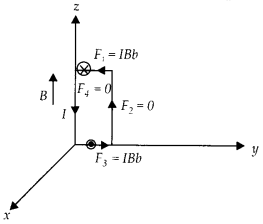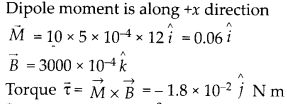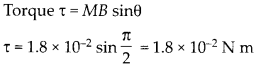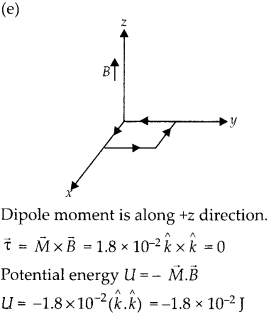Enlightened

# Question 24: NCERT Solutions for 12th Class Physics: Chapter 4-Moving Charges and Magnetism

• 0

Question 24: NCERT Solutions for 12th Class Physics: Chapter 4-Moving Charges and Magnetism

A uniform magnetic field of 3000 G is established along the positive z-direction. A rectangular loop of sides 10 cm and 5 cm carries a current of 12 A. What is the torque on the loop in the different cases shown in figure? What is the force on each case? Which case corresponds to stable equilibrium?Share

1. Solution:
(a) Let us detail each case separately,So, torque is 1.8 × 10-2 N-m along -y direction net force on the coil is zero coil not in equilibrium.
(b) Dipole moment is along +x direction

So, torque is 1.8 x 10-2 N m along -y direction. Net force on the coil is zero, coil is not in equilibrium
(c) Dipole moment is along -y directionNet force on the coil is zero, coil is not in equilibrium.
(d)

Dipole moment is at an angle 150° with the +x direction.At an angle 240° with the +x direction net force on the coil is zero, coil is not in equilibrium.Negative energy shows equilibrium is stable,
(f) Dipole moment is along – z directionPositive energy shows equilibrium is unstable.

Check the complete chapter with solutions.

NCERT Solutions for 12th Class Physics: Chapter 4-Moving Charges and Magnetism

• 0